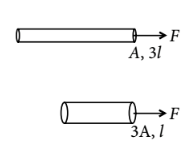Stress and strain
Question

# Two wires are made of the same material and have the same volume. The first wire has cross-sectional area A and the second wire has cross-sectional area 3A. If the length of the first wire is increased by $∆$l on applying a force F, how much force is needed to stretch the second wire by the same amount?

Moderate
Solution

## Since initial volume of wires are same Their areas of cross sections are A and 3 A  and lengths are 3l and l respectively.For wire 1,$\frac{{F}^{\text{'}}}{3A}=Y\frac{\Delta l}{l}$From the equations (i) and (ii)$\Delta l=\left(\frac{F}{AY}\right)3l=\left(\frac{{F}^{\text{'}}}{3AY}\right)l⇒{F}^{\text{'}}:$

Get Instant Solutions